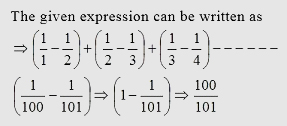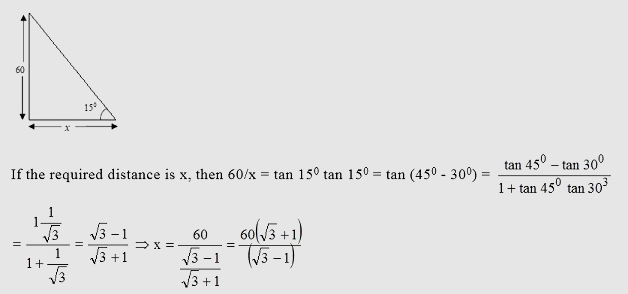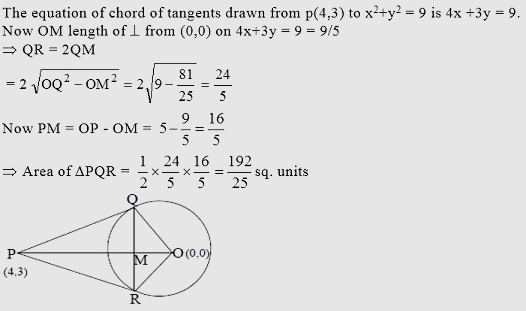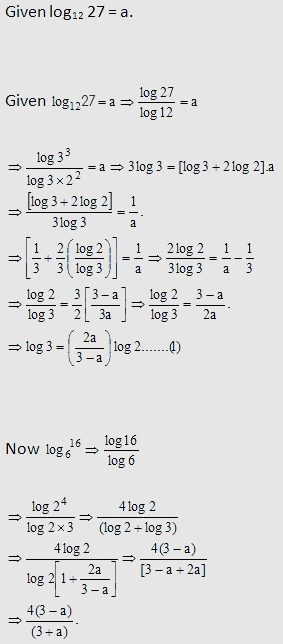# Sample Aptitude Questions of Atos

1. A toothpaste manufacturer is giving two different offers on its 100 g tube. The first one is giving 30% extra at the same price, and the second one is giving 30% off on the marked price. By what percentage is the first offer costlier than the second one?
1. 4.2%
2. 2.4%
3. 9.89%
4. 0%
Suppose the price of the toothpaste is Rs. 10. In the first offer, the price per gram would be Rs. 10/130 while in the second offer, the price per gram would be Rs. 7/100.
So the first offer is costlier than the second offer by 10/130 – 7/100 = Rs. 9/1300. Hence the first offer is costlier than the second one by 9/1300 ÷ 7/100 × 100 = 9.89%.
1. A covered wooden box has the inner measures as 115 cm, 75 cm and 35 cm and the thickness of wood is 2.5 cm. Find the volume of the wood.
1. 82125 cu. Cm
2. 81775 cu. Cm
3. 81000 cu. Cm
4. None of the above
Required volume = Outer volume of the box - Inner volume = (120 × 80 × 40) – (115 × 75 × 35) = 82125 cu. cm.
2. Find the sum of the following series :1. 100/101
2. 99/101
3. 1/101
4. 1/1003. From the top of a light house 60 meters high with its base at the sea level, the angle of depression of a boat is 15°. The distance of the boat from the foot of the light house is (in m)
1. (√3-1)/(√3+1) × 60
2. (√3+1)/(√3-1) × 60
3. (√3+1)/(√3-1)
4. (√3-1)/(√6-1)4. The area of the triangle formed by the tangents from the point (4,3) to the circle x2 + y2 = 9 and the line joining their points of contact is:
1. 25/192 square units
2. 192/25 square units
3. 385/25 square units
4. 185/25 square units1. The Hussain Sagar Express travels from Mumbai to Hyderabad. Along the way the train stops at 18 different places. So, in total, there are 20 stops including stop at Mumbai and Hyderabad. How many different tickets can be given out by the railways?
1. 190 different tickets
2. 380 different tickets
3. 145 different tickets
4. 120 different tickets
As there are 20 stations in total, so number of tickets = 20P2 = 380.
2. If log1227 = a, the log616 is
1. (3-a)/4(3+a)
2. (3+a)/4(3-a)
3. 4(3+a)/(3-a)
4. 4(3-a)/(3+a)3. Sea water contains 5% salt by weight. How many kilograms of fresh water must be added to 40 kg of sea water so that the salt content of the solution becomes 2%?
1. 60 kg
2. 50 kg
3. 40 kg
4. 55 kg
5% salt by weight means 2 kgs out of 40 kgs is salt.
Let x be the total amount of mixture
Therefore, 2% of x = 2 → x = 100 kg
So the amount of fresh water to be added in the mixture is 100 – 40 = 60 kg
4. A spherical ball 6 cm in diameter is melted and recast into 3 smaller spherical balls. The radius of the two of the there are 1.5 cm and 2 cm respectively. The radius of the third is
1. 3.5cm
2. 3.0cm
3. 2.0cm
4. 2.5cm